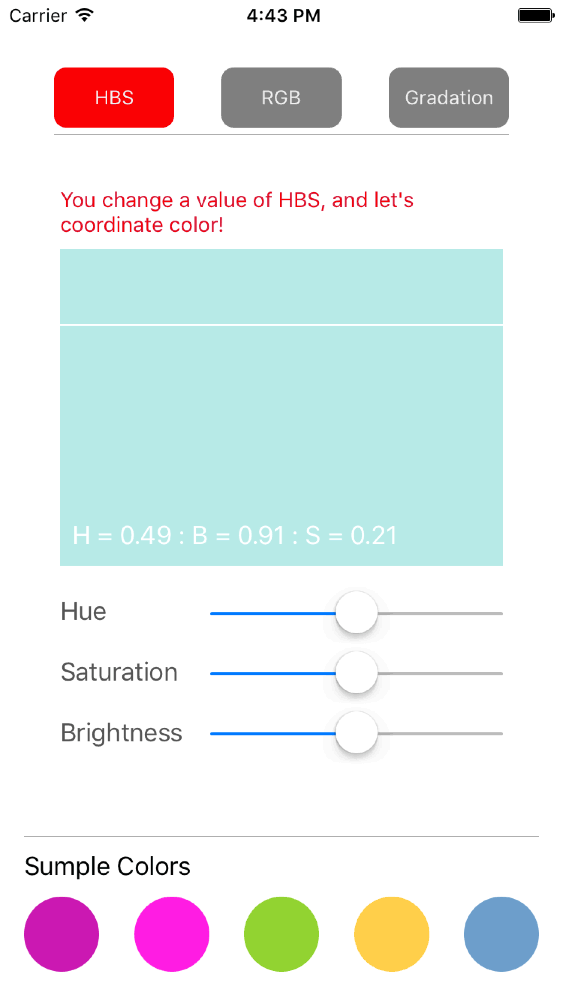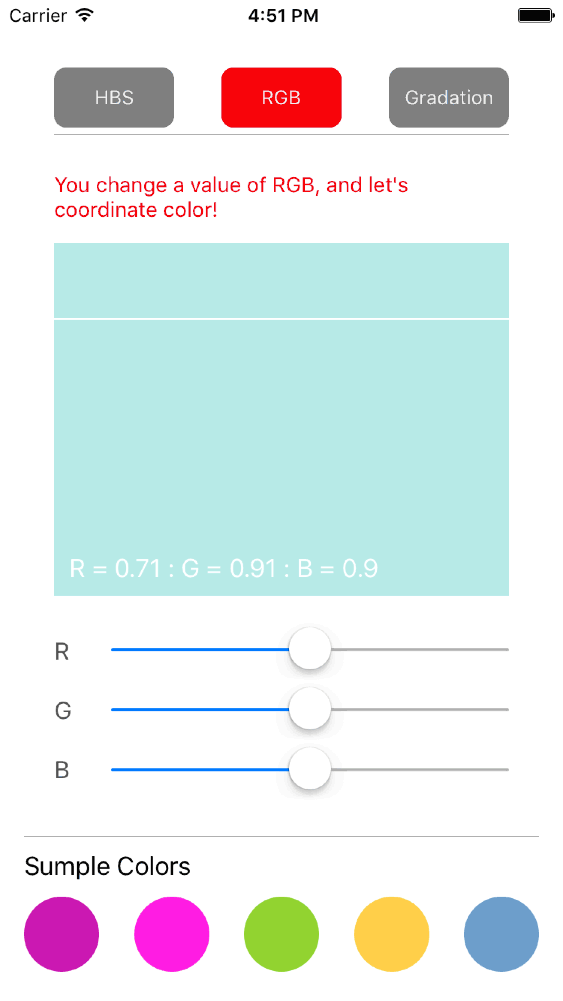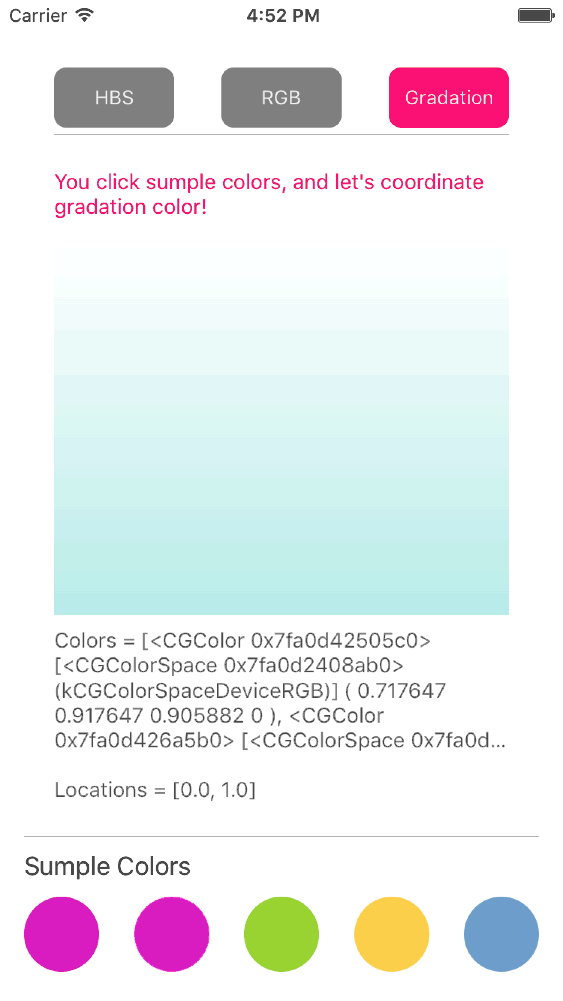Select Page
Latest 1.1.2 https://github.com/ikemai/ColorAdjuster MIT ios 8.0, requires ARC UIKit ikemaiColorAdjuster is the library which can create a new color by HBS or RGB for the cause in base color, and create gradation view.

### Demo

• UIColor can regulate numerical value of HBS and RGB of the target color.• You can coordinate gradation colors with the number of any colors.## Usage

To run the example project, clone the repo, and run `pod install` from the Example directory first.

## Requirements

• swift 2.0, iOS8.0 ~

## Installation

ColorAdjuster is available through CocoaPods. To install

``pod "ColorAdjuster"``

## Example

• Appoint it in hexadecimal and create UIColor.
``let color = UIColor(hex: 0xB7EAE7)``

# HBS

• Ajustment color of HBS.
``````let adjustmentColor = color.colorWithHBSComponent(hue: hueValue, brightness: brightnessValue, saturation: saturationValue)
• Get HBS value of color.
``````if let hbs = adjustmentColor?.colorHBS() {
print("Hue = (hbs.hue)")
print("Bridhtness = (hbs.brightness)")
print("Saturation = (hbs.saturation)")
}``````

# RGB

• Ajustment color of RGB.
``````let adjustmentColor = color.colorWithRGBComponent(red: rValue, green: gValue, blue: bValue)
• Get RGB value of color.
``````if let rbg = adjustmentColor?.colorRGB() {
print("Red = (rbg.red)")
print("Green = (rbg.green)")
print("Blue = (rbg.blue)")
}``````

``````let targetView = UIView(frame: frame)
let colors: [CGColor] = [UIColor.redColor().CGColor, UIColor.magentaColor().CGColor]

Appoint locations

``````let locations: [CGFloat] = [0.0, 1.0]Appoint startPoint and endPoint

``````let startPoint = CGPointMake(1, 0.5)
let endPoint = CGPointMake(0, 0.5)Appoint Angle = Zero

``targetView.gradientLayer.insertLayerVerticallyGradient(colors: colors, angle: .Zero)``Appoint Angle = FortyFive

``targetView.gradientLayer.insertLayerVerticallyGradient(colors: colors, angle: .FortyFive)``Appoint Ninety

``targetView.gradientLayer.insertLayerVerticallyGradient(colors: colors, angle: .Ninety)``## Function

• Appoint it in hexadecimal and create UIColor.
``public convenience init(hex: Int, alpha: CGFloat = 1)``
• UIColor ajustment color of HBS.
``public func colorWithHBSComponent(hue hue: CGFloat, brightness: CGFloat, saturation: CGFloat) -> UIColor?``
• UIColor get HBS value of color.
``public func colorHBS() -> ColorAdjuster.HBSProperties?``
• UIColor ajustment color of RGB.
``public func colorWithRGBComponent(r r: CGFloat, g: CGFloat, b: CGFloat) -> UIColor?``
• UIColor get RGB value of color.
``public func colorRGB() -> ColorAdjuster.RGBProperties?``
``````public func insertLayerVerticallyGradient(colors colors: [CGColor], locations: [CGFloat])
``````

## Properties

• Value of HBS
``````public struct HBSProperties {
public var hue: CGFloat = 0
public var brightness: CGFloat = 0
public var saturation: CGFloat = 0
public var alpha: CGFloat = 1
}``````
• Value of RGB
``````public struct RGBProperties {
public var r: CGFloat = 0
public var g: CGFloat = 0
public var b: CGFloat = 0
public var alpha: CGFloat = 1
}``````

## Author

ikemai

### Latest podspec

```{
"version": "1.1.2",
"summary": "ColorAdjuster is the library which can create a new color by HBS or RGB for the cause in base color, and create gradation view.",
"type": "MIT",
},
"authors": {
"ikemai": "[email protected]"
},
"platforms": {
"ios": "8.0"
},
"source": {